Win up to 100% scholarship on Aakash BYJU'S JEE/NEET courses with ABNAT Win up to 100% scholarship on Aakash BYJU'S JEE/NEET courses with ABNAT

# Second Law Of Thermodynamics

The second law of thermodynamics put restrictions upon the direction of heat transfer and achievable efficiencies of heat engines. The first law of thermodynamics states that the energy of the universe remains constant, though energy can be exchanged between system and surroundings, it can’t be created or destroyed.

While the first law of thermodynamics gives information about the quantity of energy transfer as a process, it fails to provide any insights about the direction of energy transfer and the quality of the energy. The first law cannot indicate whether a metallic bar of uniform temperature can spontaneously become warmer at one end and cooler at others. All that the law can state is that there will always be energy balance if the process occurs. It is the second law of thermodynamics that provides the criterion for the feasibility of any process. A process cannot occur unless it satisfies both the first and second laws of thermodynamics.

## What is the Second Law of Thermodynamics?

The second law of thermodynamics states that any spontaneously occurring process will always lead to an escalation in the entropy (S) of the universe. In simple words, the law explains that an isolated system’s entropy will never decrease over time.

Nonetheless, in some cases where the system is in thermodynamic equilibrium or going through a reversible process, the total entropy of a system and its surroundings remains constant. The second law is also known as the Law of Increased Entropy.

### Second Law of Thermodynamics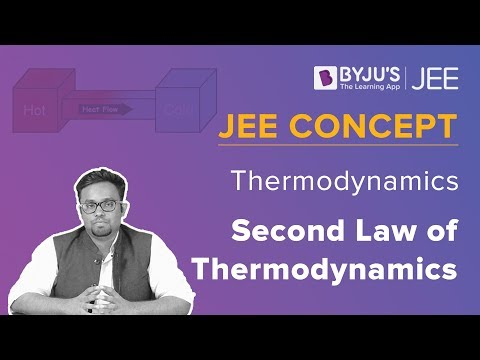The second law clearly explains that it is impossible to convert heat energy to mechanical energy with 100 per cent efficiency. For example, if we look at the piston in an engine, the gas is heated to increase its pressure and drive a piston. However, even as the piston moves, there is always some leftover heat in the gas that cannot be used for carrying out any other work. Heat is wasted and it has to de discarded. In this case, it is done by transferring it to a heat sink or in the case of a car engine, waste heat is discarded by exhausting the used fuel and air mixture to the atmosphere. Additionally, heat generated from friction that is generally unusable should also be removed from the system.

## Second Law of Thermodynamics Equation

Mathematically, the second law of thermodynamics is represented as;

ΔSuniv > 0

where ΔSuniv is the change in the entropy of the universe.

Entropy is a measure of the randomness of the system or it is the measure of energy or chaos within an isolated system. It can be considered as a quantitative index that describes the quality of energy.

Meanwhile, there are few factors that cause an increase in entropy of the closed system. Firstly, in a closed system, while the mass remains constant there is an exchange of heat with the surroundings. This change in the heat content creates a disturbance in the system thereby increasing the entropy of the system.

Secondly, internal changes may occur in the movements of the molecules of the system. This leads to disturbances which further causes irreversibilities inside the system resulting in the increment of its entropy.

Also Read: Third Law of Thermodynamics

## Different Statements of The Law

There are two statements on the second law of thermodynamics which are;

1. Kelvin- Plank Statement
2. Clausius Statement

### Kelvin-Planck Statement

It is impossible for a heat engine to produce a network in a complete cycle if it exchanges heat only with bodies at a single fixed temperature.

Exceptions:

If Q2 =0 (i.e., Wnet = Q1, or efficiency=1.00), the heat engine produces work in a complete cycle by exchanging heat with only one reservoir, thus violating the Kelvin-Planck statement.

Also Read: Zeroth Law Of Thermodynamics

### Clausius’s Statement

It is impossible to construct a device operating in a cycle that can transfer heat from a colder body to a warmer one without consuming any work. Also, energy will not flow spontaneously from a low-temperature object to a higher temperature object. It is important to note that we are referring to the net transfer of energy. Energy transfer can take place from a cold object to a hot object by transfer of energetic particles or electromagnetic radiation. However, the net transfer will occur from the hot object to the cold object in any spontaneous process. And some form of work is needed to transfer the net energy to the hot object.  In other words, unless the compressor is driven by an external source, the refrigerator won’t be able to operate. The heat pump and refrigerator work on Clausius’s statement.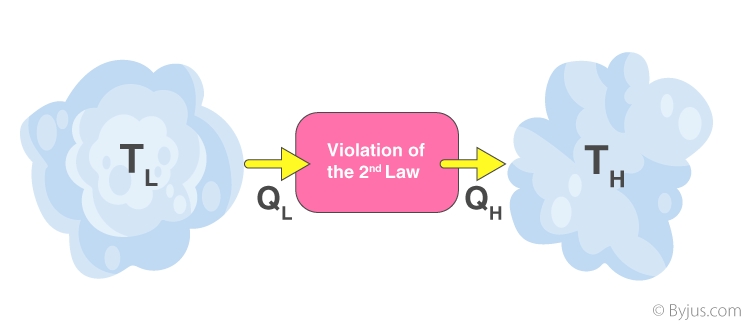Both Clausius’s and Kelvin’s statements are equivalent i.e a device violating Clausius’s statement will also violate Kelvin’s statement and vice versa.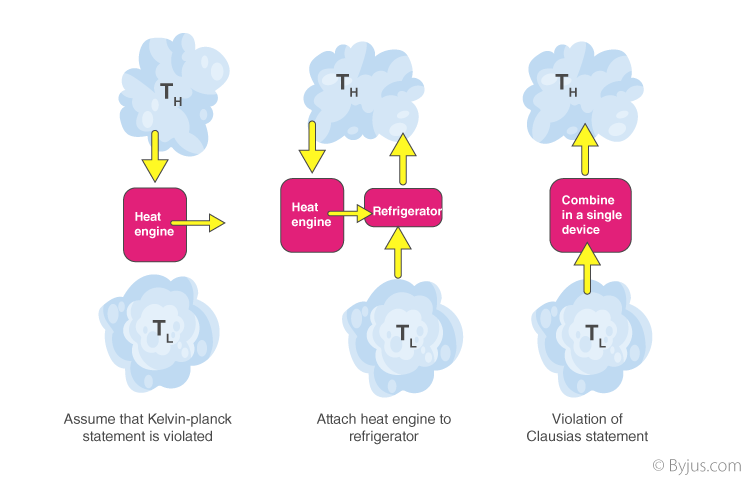In addition to these statements, a French physicist named Nicolas Léonard Sadi Carnot also known as the “father of thermodynamics,” basically introduced the Second Law of Thermodynamics. However, as per his statement, he emphasized the use of caloric theory for the description of the law. Caloric (self repellent fluid) relates to heat and Carnot observed that some caloric was lost in the motion cycle.

## Perpetual Motion Machine of the Second Kind (PMM2)

The device that produces work while interacting with a single heat reservoir is known as a perpetual motion machine of the second kind (PMM2). Also, a device that violates the second law of thermodynamics is a perpetual motion machine of the second kind.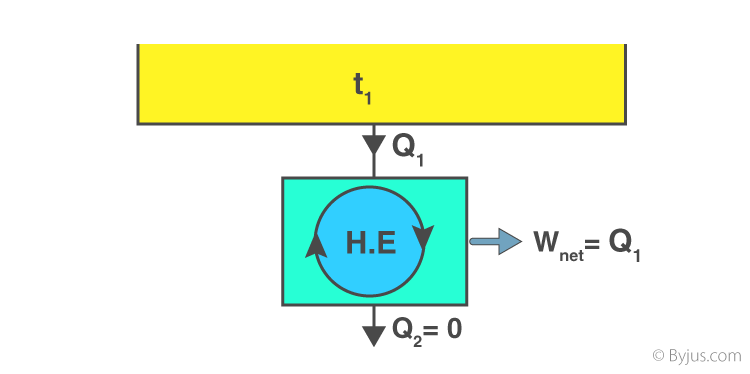Thus, a heat engine has to interact with at least two thermal reservoirs at different temperatures to produce work in a cycle. So long as there is a difference in temperature, motive power (i.e., work) can be produced. If the bodies with which the heat engine exchanges are of finite heat capacities, work will be produced by the heat engine until the temperature of the two bodies is equalised.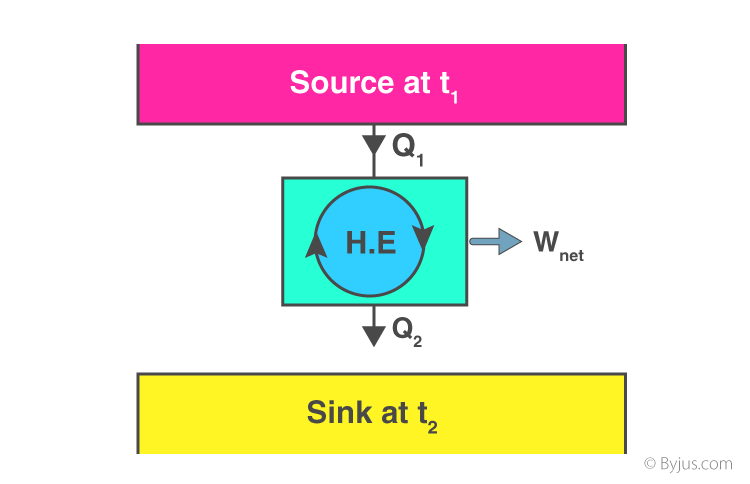## Solved Examples of Second Law Of Thermodynamics

1. A heat pump uses 300 J of work to remove 400 J of heat from the low-temperature reservoir. How much heat is delivered to a higher temperature reservoir?

Solution:

W = 300 J

Q= 400 J

QH = WQC

QH = 300 J + 400 J

QH = 700 J

Heat delivered to the higher temperature reservoir is 700 J.

2. A reversible heat engine receives 4000 KJ of heat from a constant temperature source at 600 K. If the surrounding is at 300K then determine (a) the availability of heat energy (b) unavailable heat.

Solution:

Q1 = 4000 KJ

T1 = 600K

T0 = 300 K

$$\begin{array}{l}Change in entropy\left ( \Delta S \right ) = \int \frac{dQ}{T}\end{array}$$
$$\begin{array}{l}\Delta S = \frac{Q_{1}}{\left ( T_{1}+T_{0} \right )}\end{array}$$

Change in entropy = 4.44 KJ/K

The availability of heat energy,

A = Q1 – T0(ΔS)

A = 4000 – 300(4.44)

A = 2,668 KJ.

Unavailable heat (U.A) = T0 (ΔS)

(U.A) = 300 (4.44)

(U.A) = 1332 KJ.

(a) the availability of heat energy (A) = 2668 KJ

(b)Unavailable heat (U.A) = 1332 KJ

### Thermodynamics Important Topics### Thermodynamics Important Questions### Thermodynamics Top 7 Questions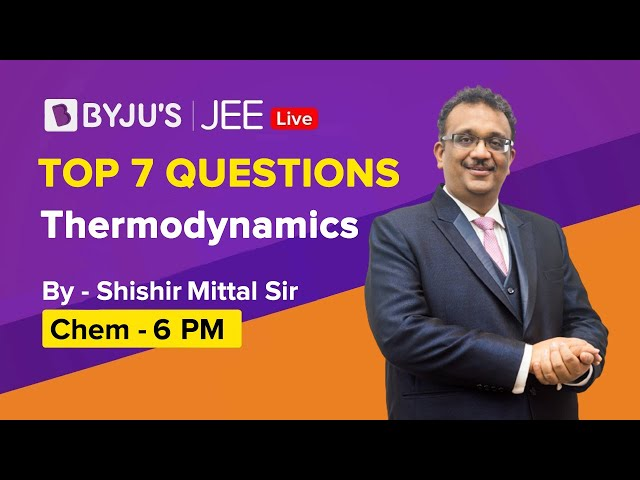### Thermodynamics JEE Solutions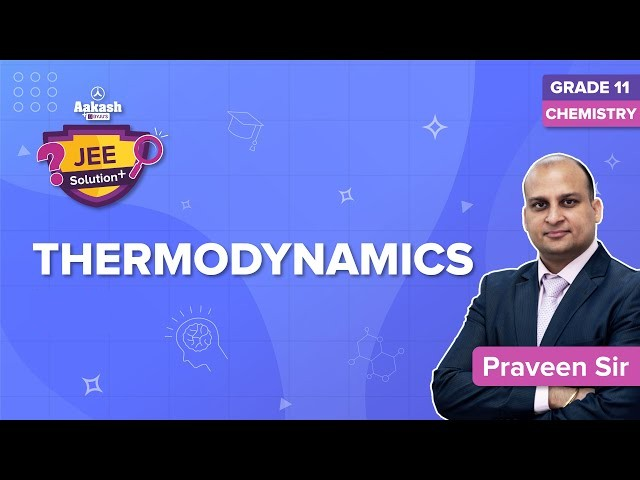### Thermodynamics – Full Chapter Revision### Thermodynamics – Top 15 Most Important and Expected JEE Main Questions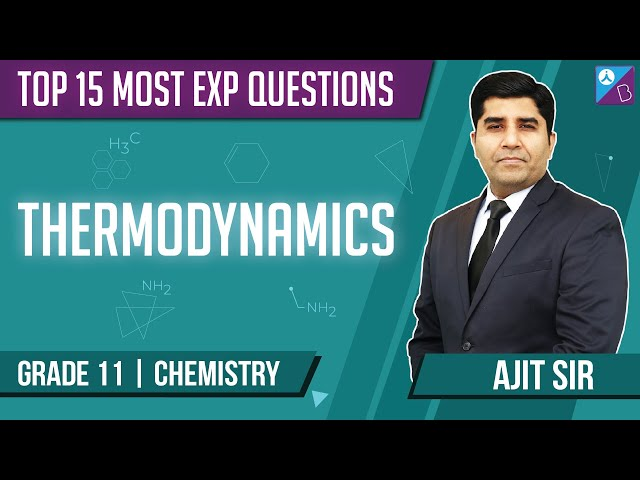### Thermodynamics JEE Main Important Questions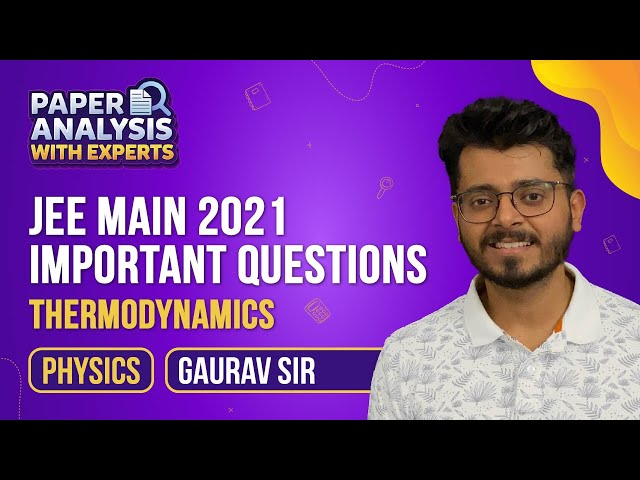## Frequently Asked Questions on Second Law of Thermodynamics

### What is Kelvin’s statement of the second law of thermodynamics?

According to the second law of thermodynamics by Kelvin, it is impossible to get a continuous supply of work by cooling a body to a temperature lower than that of the coolest of surroundings.

### What is Clausius’ statement of the second law of thermodynamics?

It is impossible for a self-acting machine unaided by an external agency to heat from one body at a lower temperature to another body at a higher temperature.

### When is there a violation of Kelvin’s statement of the second law?

Suppose we have an engine which gives a continuous supply of work when it is cooled below the temperature of the surroundings. This is a violation of the Kelvin statement.

### Give one application of the second law of thermodynamics.

The second law of thermodynamics is applied in refrigerators.

### How is entropy related to the second law of thermodynamics?

According to the second law of thermodynamics, the total entropy of the system will either increase or remain constant, it will never decrease.

### Will entropy decrease?

Entropy will not decrease. Entropy will increase for an irreversible process and will be zero for a reversible process.

### What factors influence entropy?

A substance’s entropy rises with its molecular weight and complexity, as well as with temperature. As the pressure or concentration decreases, the entropy increases.

### List a few properties of entropy.

(1) It only depends on the mass of the system since it is an extensive quantity.
(2) There is an increase in the entropy of the universe.
(3) Entropy is never zero.
(4) For an adiabatic thermodynamic system, the entropy will remain constant.
(5) Entropy and temperature are inversely proportional.
(6) In a cyclic process, there is no change in state. Therefore, the entropy remains constant.
(7) For an irreversible process, entropy increases.
(8) For reversible processes, entropy decreases.

Test Your Knowledge On Second Law Of Thermodynamics!